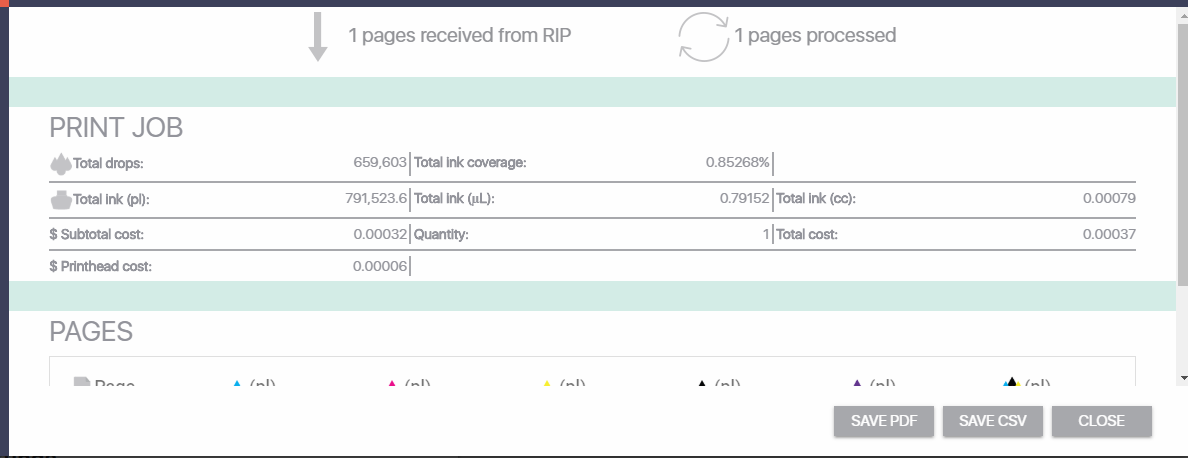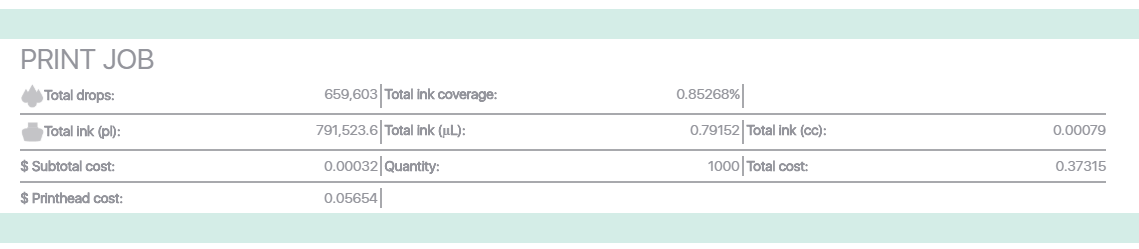5/31/18

There was a problem with how we calculated printhead usage that has now been resolved.

Previously, jobs with a copy count of 1 were all calculated correctly.  However, jobs with a  copy count higher than 1 were undercalculated in terms of printhead usage.  Ink calculations have always been correct and have not changed.  However, printhead usage calculations are now changed and corrected.

Using an example label job we have the unchanged calculation for quantity of 1.

The printhead usage cost is some small fraction of a cent.Here was the quantity of 1000.  Instead of 37 cents, the cost shows 32 cents.  This is because we calculated the printhead usage cost for a quantity of one.  Only the ink was calculated at the quantity of 1000.Here is the job calculated today at a quantity of 1000.  Now a quantity of 1000 labels is calculated at 37 cents because the printhead cost was correctly calculated.